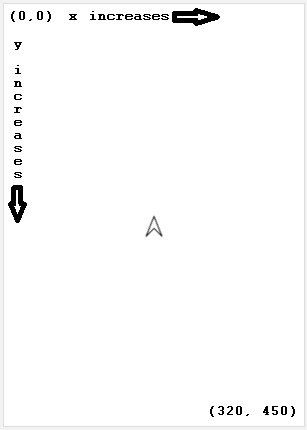# moveTo

Category:Turtle

## moveTo(x, y)

Category: Turtle

Moves the turtle to a specific (x,y) position on the screen.

Use moveTo(x,y) when drawing a picture where parts of the picture need to be at very specific positions on the screen. Unlike move(x,y), which moves the turtle relative to it's current position, moveTo(x,y) moves the turtle to an absolute position on the screen. The direction that the turtle is facing remains unchanged.

### Examples

```// Move the turtle near the top, left of the screen.
moveTo(50, 50);
```

Example: Square Draw a square by connecting the four corners in counterclockwise order.

 ```// Draw a square by connecting the four corners in counterclockwise order. penUp(); moveTo(50, 50); penDown(); moveTo(50, 270); moveTo(270, 270); moveTo(270, 50); moveTo(50, 50); ```Example: Parabola Draw an half parabola opening downward.

```// Draw an half parabola opening downward.
penUp();
for (var x = 0; x < 200; x++) {
var y = x*x/100;
moveTo(x,y);
penDown();
}
```

### Syntax

```moveTo(x, y);
```

### Parameters

Name Type Required? Description
x number Yes The x coordinate on the screen to move the turtle to.
y number Yes The y coordinate on the screen to move the turtle to.

### Returns

No return value. Moves turtle only.

### Tips

• Use penUp() before calling moveTo(x,y) to have the turtle not draw as it moves.
• (0,0) is upper left corner and x increases from left to right and y increases from top to bottom.
• The screen default size is 320 pixels wide and 450 pixels high, but you can move the turtle off the screen by exceeding those dimensions.
• There are three ways to move the turtle in a straight line:
• Specify the number of pixels to move the turtle in the direction it is facing using moveForward(pixels) or moveBackward(pixels).
• Specify a number of pixels in the x and y direction to move the turtle using move(x,y), regardless of direction that the turtle is facing.
• Specify an x and y pixel location on the screen to move the turtle to using moveTo(x,y), regardless of direction that the turtle is facing.Found a bug in the documentation? Let us know at documentation@code.org

Found a bug in the documentation? Let us know at documentation@code.org